• 阶次已知的线性系统差分方程模型的参数辨识；阶次未知的线性系统差分方程模型的参数辨识的MATLAN程序＋仿真图片
• 线性系统稳定性分析的MATLAB方法 ;线性系统稳定的充分必要条件 线性系统的稳定性仅取决于系统自身的固有特性而与外界条件无关 ;代数法;根轨迹法判断系统稳定性;奈奎斯特图法判断系统稳定性;波特图法判断系统稳定性;1...
• 在文 中, 求解线性定常系统状态方程时, 计算冗长烦琐, 十分不便。本文以最新版MATLAB6.5 为基础, 给出应用MATLAB 求解状态方程的两种实现方法.为了方便使用, 这两种方法被分别编制成MATLAB 的函数形式, 求解状态方程...
• 方法 精确法 近似法 精确法 原理 推导 结伦 近似法
方法
精确法近似法
精确法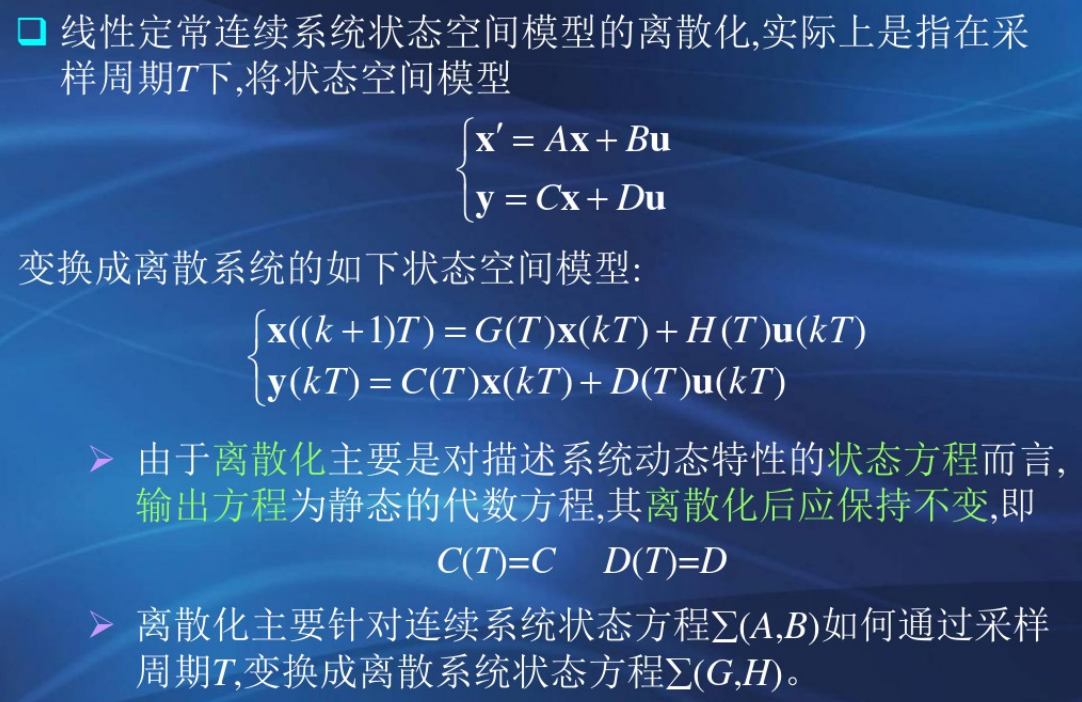原理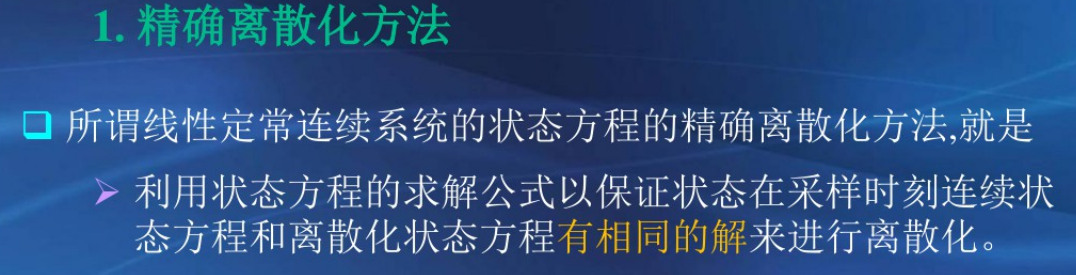推导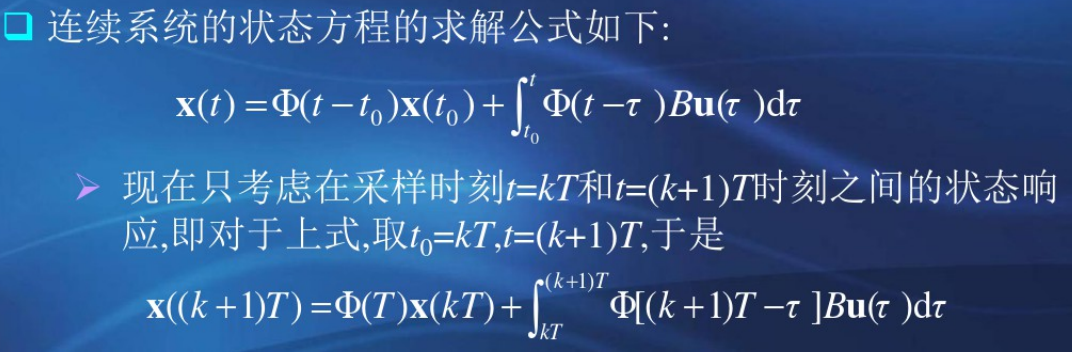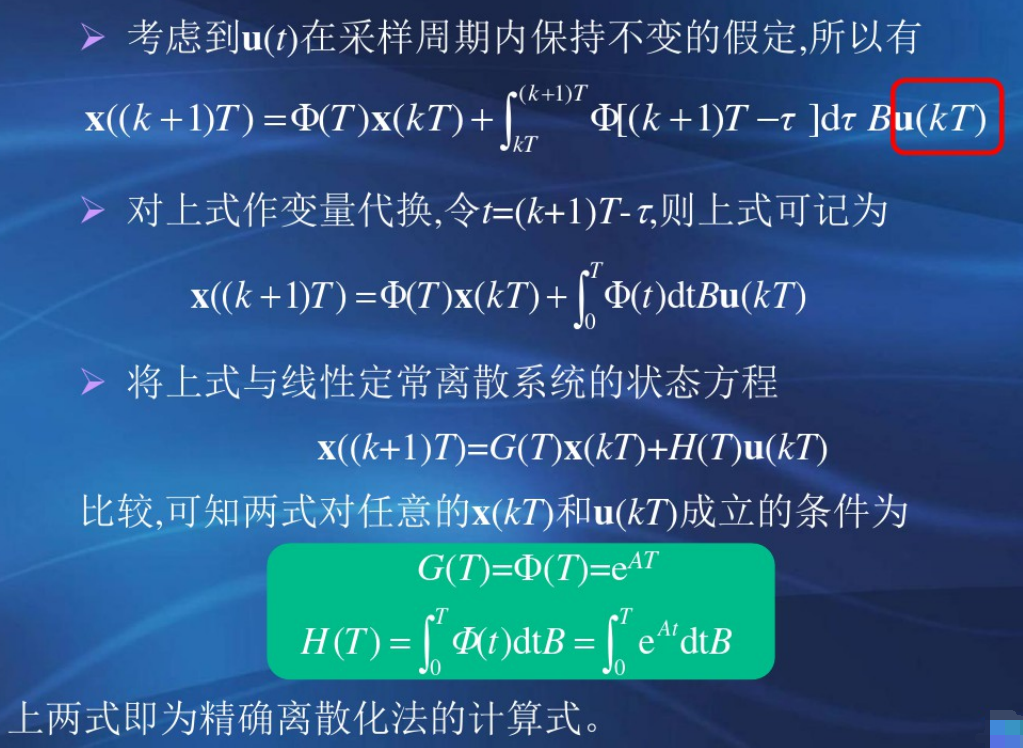结伦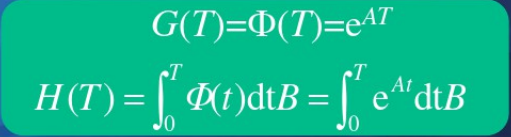近似法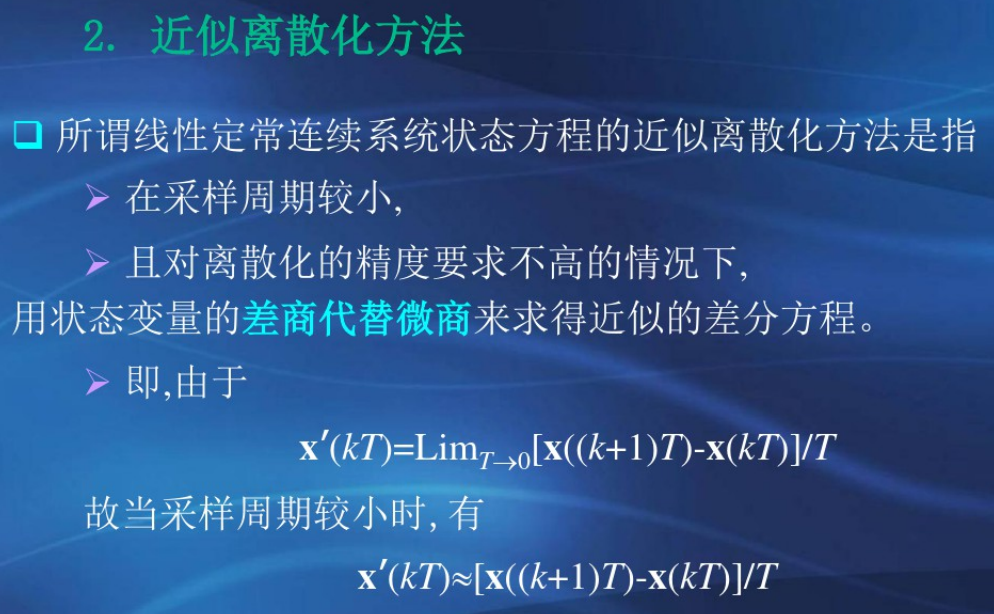展开全文• 介绍了分数阶线性定常系统的能控性定义以及状态方程解的一般表达式,给出了状态转移矩阵的概念,并在此基础上提出并证明了分数阶线性定常系统能控性的格拉姆(Gram)矩阵判据,所得结论对分数阶控制系统的分析与综合是...
• 在《自动控制原理》（胡寿松著第六版）这本书中的第12页，讲到了自动控制系统的分类，其中本书主要的研究的是线性定常系统，见图 但第一次见到它的表达式难免产生疑问：什么是线性微分方程？为什么线性定常...
注明：本文部分内容摘自知乎的某些问题的某些回答，由于比较庞杂，故不一一标明出处，但是会推荐一些值得大家学习的答主，说不定他的某个回答或文章就能帮到你
--------------------------------------------------------------------正文-----------------------------------------------------------------------
在《自动控制原理》（胡寿松著第六版）这本书中的第12页，讲到了自动控制系统的分类，其中本书主要的研究的是线性定常系统，见图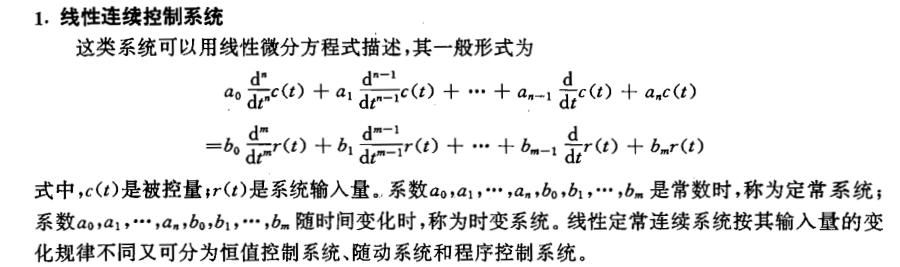但第一次见到它的表达式难免产生疑问：什么是线性微分方程？为什么线性定常系统要用线性微分方程表示？
下面是几个比较容易理解且相我认为正确的回答这位大神的文章都很好，感兴趣的可以搜一搜，看文章又不要钱，这里为了解决当前问题只截选了相关部分
你们如果懒得搜，这是他的知乎主页链接https://www.zhihu.com/people/galieluo/activities

要研发一个成功的机电产品，在物理实体上需要解决什么问题呢？——以机器人为例（也是一个典型的机电产品），作为一个“人”，必须要解决好两个“流”的问题：一个是“信息流”，另一个是“动力流”。我们经常说的自动控制，信号处理、传感检测其实都属于“信息流”的范畴，解决的是大脑和神经的问题。
而“动力流”，则是要解决躯干和肌肉问的题。“动力流”包括动力的产生、传递和执行，产生动力（机械能）的源泉一般是发动机（engine）或者是电动机（motor），一个是由化学能转化而来，一个是由电能转化而来。传动机构主要包括各种杆系、齿轮、滚珠丝杠、轴承等部件组成，部件之间又通过旋转运动副、直线运动副等连接。执行机构典型的如机械手等。
数学上怎么解决两个“流”的事呢？——两类微分方程：一个是常微分方程(Ordinary Differential Equation)简称ODE；另一个是偏微分方程(Partial Differential Equation)简称PDE。有的童鞋可能纳闷啦？我们学的方程那么多，为啥必须是微分方程呢？——答案很简单，因为我们要研究的系统都是变化的，而变化也就意味着微分，如果一时不能理解，想一想微分的定义——函数改变量的线性主要部分。
一个典型的常微分方程长成下面这个样子：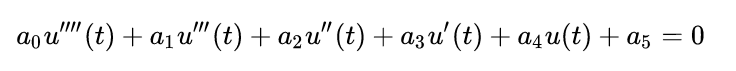变量只和时间 t 有关系，和空间位置没关系，换句话说，常微分方程描述的是单质点的变化规律，如：某个物体在重力作用下做自由落体运动，下落距离随时间变化的规律；火箭在发动机推动下在空间飞行，飞行的轨道等等。常微分方程一般是把研究对象当成一个质点或者刚体，研究整体的运动规律。
或者说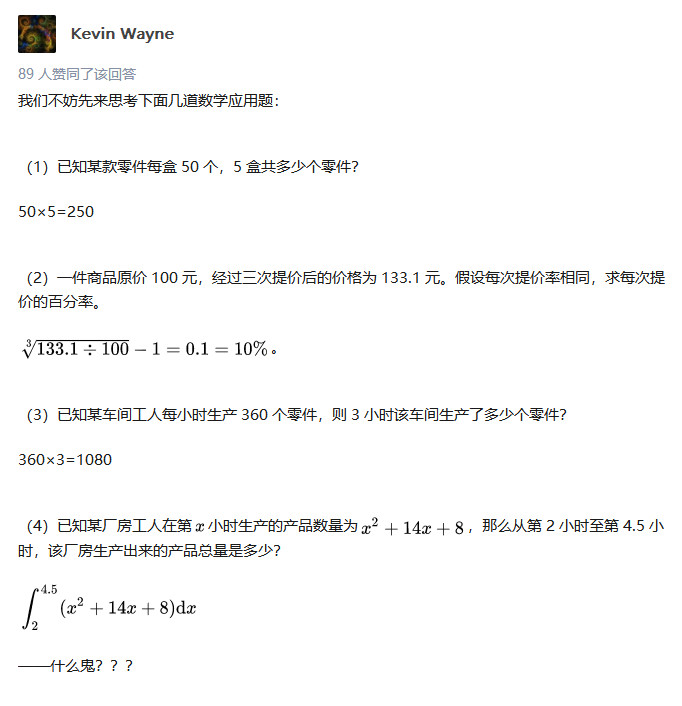偷个懒直接截图了，这样也方便你们找到出处
虽然到这可能还是对微分方程一知半解，但应该对微分方程的意义有了一个大概的了解了
展开全文• 控制系统 状态空间表达式 的 解 就是求解系统： x˙=Ax+Bux(0)=x0y=Cx+Du \begin{aligned} \dot{x}&=Ax+Bu \qquad x(0)=x_{0}\\ y&=Cx+Du \end{aligned} x˙y​=Ax+Bux(0)=x0​=Cx+Du​ 在给定初始条件x(0)=...
将这句话拆分开： 控制系统 状态空间表达式 的 解
就是求解系统：

x

˙

=

A

x

+

B

u

x

(

0

)

=

x

0

y

=

C

x

+

D

u

\begin{aligned} \dot{x}&=Ax+Bu \qquad x(0)=x_{0}\\ y&=Cx+Du \end{aligned}

在给定初始条件

x

(

0

)

=

x

0

x(0)=x_{0}

和控制输入

u

(

t

)

u(t)

共同作用下
状态向量和输出向量的随时间变化的规律

x

(

t

)

x(t)

和

y

(

t

)

y(t)

1.线性系统一定满足叠加原理：
系统在初始状态

x

0

x_0

和控制输入

u

(

t

)

u(t)

共同作用下的运动状态

x

(

t

)

x(t)

，可以分解为由初始状态

x

0

x_0

和控制输入

u

(

t

)

u(t)

分别单独作用产生的运动状态

x

0

u

(

t

)

x_{0u}(t)

和

x

0

x

(

t

)

x_{0x}(t)

的叠加，即

x

(

t

)

=

x

0

u

(

t

)

+

x

0

x

(

t

)

{\color{00a100}x(t)}={\color{9100c9}x_{0u}(t)}+{\color{008b8b}x_{0x}(t)}

2.零输入响应
定义为只有初始状态作用即

x

0

≠

0

x_{0} \neq 0

，而无输入作用，即

u

≡

0

u\equiv 0

是系统的状态响应

x

0

u

(

t

)

x_{0u}(t)

.
零输入响应

x

0

u

(

t

)

x_{0u}(t)

就是自治方程:

x

˙

=

A

x

,

x

(

0

)

=

x

0

\dot{x}=Ax, x(0)=x_{0}

在非平衡初始状态

x

0

x_{0}

作用下的自由解
3.零状态响应
定义为只有输入作用即

u

≢

0

u \not\equiv 0

，而无初始状态作用即

x

0

=

0

x_{0}=0

时系统的状态响应

x

0

x

(

t

)

.

x_{0x}(t).

零状态响应

x

0

x

(

t

)

x_{0x}(t)

就是方程

x

˙

=

A

x

+

B

u

x

(

0

)

=

0

在平衡初始状态时，输入

u

u

激励作用下的强迫运动
4.零输入响应计算
线性定常系统其次状态方程:

x

˙

=

A

x

,

x

(

0

)

=

x

0

,

t

≥

0

(

1

)

\dot{x}=Ax, x(0)=x_{0}, t \ge 0 \qquad(1)

的解，即系统的零输入响应

x

0

u

x_{0u}

为：

x

0

u

=

e

A

x

x

0

,

t

≥

0

式中，

e

A

t

e^{At}

为系统矩阵

A

A

的矩阵指数函数：

e

A

t

=

d

e

f

I

+

A

t

+

1

2

!

A

2

t

2

+

.

.

.

=

∑

k

=

0

∞

1

k

!

A

k

t

k

e^{At}\overset{def}{=}I+At+\frac{1}{2!}A^{2}t^{2}+...=\sum_{k=0}^{\infty}\frac{1}{k!}A^{k}t{^k}

证明：
令方程

(

1

)

(1)

的解为系数向量待定的一个幂级数，即：

x

0

u

(

t

)

=

b

0

+

b

1

t

+

b

2

t

2

+

.

.

.

=

∑

k

=

0

∞

b

k

t

k

(

2

)

其必满足方程

(

1

)

(1)

，将上式代入方程

(

1

)

(1)

，可得：

b

1

+

2

b

2

t

+

3

b

3

t

2

+

.

.

.

=

A

(

b

0

+

b

1

t

+

b

2

t

2

+

.

.

.

)

b_{1}+2b_{2}t^{}+3b_{3}t^{2}+...=A(b_{0}+b_{1}t+b_{2}t^{2}+...)

比较可得：

b

1

=

A

b

0

b

2

=

1

2

A

b

1

=

1

2

A

2

b

0

.

.

.

b

k

=

1

k

!

A

k

b

0

.

.

.

\begin{aligned} b_{1}&=Ab_{0}\\ b_{2}&=\frac{1}{2}Ab_{1}=\frac{1}{2}A^{2}b_{0} \\ ...\\ b_{k}&=\frac{1}{k!}A^{k}b_{0}\\ ... \end{aligned}

将求得的待定系数，代入式

(

2

)

(2)

可得：

x

0

u

(

t

)

=

(

I

+

A

t

+

1

2

!

A

2

t

2

+

1

3

!

A

3

t

3

.

.

.

)

b

0

x_{0u}(t)=(I+At+\frac{1}{2!}A^{2}t^{2}+\frac{1}{3!}A^{3}t^{3}...)b_{0}

有初始条件

x

0

u

(

t

)

=

x

0

x_{0u}(t)=x_{0}

可得

b

0

=

x

0

b_{0}=x_{0}

，故：

x

0

u

(

t

)

=

(

I

+

A

t

+

1

2

!

A

2

t

2

+

1

3

!

A

3

t

3

.

.

.

)

x

0

=

e

A

t

x

0

x_{0u}(t)=(I+At+\frac{1}{2!}A^{2}t^{2}+\frac{1}{3!}A^{3}t^{3}...)x_{0}=e^{At}x_{0}

证毕
5.线性定常系统零输入响应的几点说明
如果

t

t

取某个固定值，零输入响应就是状态空间中由初始状态

x

0

x_{0}

经线性变换阵

e

A

t

e^{At}

所导出的一个变换点。系统的自由运动就是由初始状态

x

0

x_{0}

出发，并由各个时刻的变换点

x

(

t

)

x(t)

所组成的一条轨线；零输入响应轨线的形态有矩阵指数函数唯一的确定；线性定常系统渐进稳定的充要条件是：

lim

⁡

x

→

+

∞

e

A

t

=

0

\lim_{x\rightarrow +\infty}e^{At}=0

系统渐进稳定的条件是：当

x

→

+

∞

x\rightarrow +\infty

，自由运动的轨线将趋于系统的平衡状态

x

e

=

0

x_{e}=0

，即状态空间的原点求解零输入响应的核心是计算矩阵指数函数

e

A

t

e^{At}

当

x

(

t

0

)

=

x

0

,

t

0

≠

0

x(t_{0})=x_{0}, t_{0}\neq0

时，零输入响应表达式更一般的形式:

x

0

u

=

e

A

(

t

−

t

0

)

x

0

t

≥

t

0


展开全文• 通过分析系统性质及矩阵A、B的特点，提出了两种新的有效的多输入线性定常系统闭环极点配置的算法.
• 给定初始状态为零的线性定常系统: x˙=Ax+Bu，x(0)=0，t≥0 \dot x=Ax+Bu，x(0)=0，t\ge0 x˙=Ax+Bu，x(0)=0，t≥0 其中，xxx为nnn维状态向量，uuu为rrr维输入向量，AAA和BBB分别为n×n\timesn×n和n×rn\times rn×...
零状态响应计算
给定初始状态为零的线性定常系统:

x

˙

=

A

x

+

B

u

，

x

(

0

)

=

0

，

t

≥

0

\dot x=Ax+Bu，x(0)=0，t\ge0

其中，

x

x

为

n

n

维状态向量，

u

u

为

r

r

维输入向量，

A

A

和

B

B

分别为

n

×

n\times

n和

n

×

r

n\times r

的常阵，那么系统的零状态响应可以表示为：

x

0

x

(

t

)

=

∫

0

t

e

A

(

t

−

τ

)

B

u

(

τ

)

d

τ

t

≥

0

(累了累了，休息一会儿…)
展开全文• 摘　要：利用线形定常系统能观性的格拉姆判据及Lyapunov稳定性理论，讨论线性定常最优反馈系统的稳定性问题。 关键词：线性定常系统；最优控制反馈系统；稳定性；Lyapunov1　问题的提出　已知线性定常系统和二次型...稳定性 Lyapunov
• 线性系统理论中,渐近稳定性判据占有重妥的地位,目前常用的四种据为 Routh-Hurwitz 4判断,连分式Hurwitz行列据和Lienard-Chipart判据 ,本文通过引入一个可实现的系统,揭示了四种-PJ据间的内在联系,给出了这四种荆据...
• 文章目录判别线性系统与非线性系统直观判别法线性系统与非线性系统的区别判别时变与时不变系统 判别线性系统与非线性系统 直观判别法 线性系统的表达式中只有状态变量的一次项，高次、三角函数以及常数项都没有，...
• 文章目录A 时域分析法基础 ...系统的时间响应，不仅取决于系统本身的结构参数，还有系统的初始状态，以及加在系统的外作用相关。 （1）典型初始状态 在t=0–时，系统处于静止状态，即 （2）典型外作用
• 这篇文章解释了线性系统的基本方面，它在电气工程和信号处理中起着基础性的作用。 什么是系统? 先做重要的事。就我们的目的而言，系统是接受输入信号并产生输出信号的组件或组件的集合。这些组件可以是物理设备，...
• 借鉴解决线性定常系统鲁棒控制问题的思路, 把周期 问题转化为一类范数有界问题, 避开了求取系统状态转移矩阵; 设计了基于LMI 的使LTVP 系统镇定的无记忆反馈 控制器和基于LMI 的使LTVP 系统鲁棒镇定无...
• 论文研究-线性定常模糊随机系统的响应分析.pdf,
• 根据系统是否含有参数随时间变化的元件，自动控制系统可分为时变系统定常系统两大类。 定常系统又称为时不变系统，其特点是：系统的自身性质不随时间而变化。具体而言，系统响应的性态只取决于输入信号的性态和...
• 线性定常连续系统状态方程的解——齐次方程 1.求解 齐次状态方程：x=Ax 其解描述的是： 即无控情况下在初始状态作用下系统的自由运动 解法： 将标量齐次微分方程的解法推广到向量中 标量中： 标量微分方程： x=ax ...
• 论文研究-非整数滞后中立型线性定常离散控制大系统的镇定.pdf, 本文应用刘永清建立的频率等价法与非整数中立型线性离散系统的比较原理［２］，给出了非整数滞后中立型...
• 线性系统理论郑大钟上.线性系统理论线性系统理论郑大钟上.郑大钟上.
• 在实际系统中大量存在的常见非线性系统的性能会造成很坏的影响。主要研究不带常见非线性和带有常见非线性被控对象，在比例、积分、微分PID(Proportional Integration Differential)控制系统的参数变化规律。选取4...
•经验分享
• ## 系统辨识

万次阅读 2019-07-28 22:12:40
• 第一部分介绍了20多年来无穷维线性系统控制理论的最新发展，特别是适、正则系统的抽象理论。也讨论了可控性、可观性、能稳性、可检性、可优性、可估性、实现，以及极点配置等几个主要的基础性概念。第二部分介绍了...
• 讨论连续定常线性系统最优调节器的逆问题,得到一种求加权矩阵Q和R的简便方法.
• 线性系统可能存在多个局部渐进稳定的平衡态 在非线性系统找李雅普诺夫函数时，要通过平移，将讨论的平衡态挪到原点 其中的三种方法主要指整理第一种 克索夫斯基法/雅可比矩阵法 通过特殊函数来构造李雅普诺夫...
• 要想将信号横坐标的尺寸展宽或者压缩（称为尺度变换），可将变量at(a为非零常数)代替原信号f(t)的自变量t，得到信号f(at)。   连续信号f(t)    f(t) ----->f(at)  若|a| > 1,则以原点为基准 压缩   ......